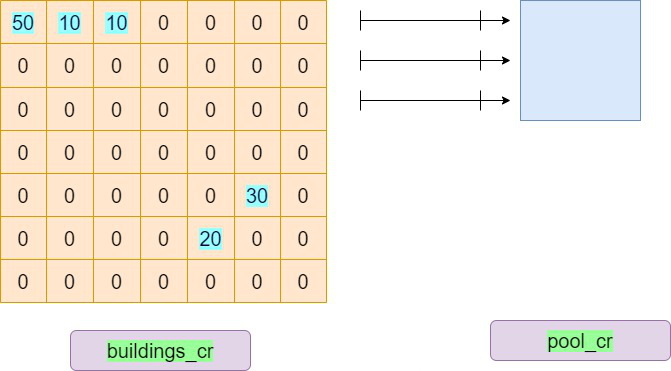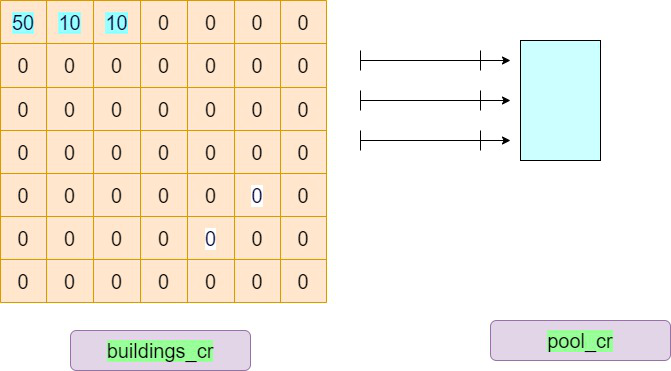# Maximum possible cost of buildings with pool view

• Last Updated : 24 Aug, 2022

Consider a series of buildings in a 2-D square plot with different heights and only those buildings are chargeable that have a clear and straight view of the pool from their building. The pool also spans a 2-D square plot with certain coordinates. Given the Coordinates of a square pool, the number of buildings, the charge being paid by customers, and the height of the building at each coordinate, the task is to find the maximum cost possible considering the fee is distributed and charged equally across all heights.

Examples:

Input: pool_coordinates = {{0, 0}, {2, 0}, {0, 2}, {2, 2}}, buildings = 5, charge =10
buildings coordinates = {{0, 0, 50}, {4, 6, 30}, {5, 5, 20}, {0, 1, 10}, {0, 2, 10}}
Output: 500
Explanation: The first three rows are chargeable
with effective pool view heights as 40, 0 and 10.5 Buildings with Charge 10

Input: pool_coordinates = {{1, 0}, {3, 0}, {1, 1}, {3, 1}}, buildings = 3, charge = 5
buildings coordinates = {{0, 0, 50}, {0, 1, 10}, {0, 2, 10}}
Output: 0
Explanation: Second, third and fourth rows are chargeable
but they don’t have any building at all.3 Buildings with Charge 5

Approach: To solve the problem follow the below idea:

The problem can be solved using the greedy approach by finding maximum heights from right to left since buildings with bigger heights will block the view of the smaller buildings. The remaining part of these buildings will then be charged equally.

Follow the given steps to solve the problem:

• Find the maximum coordinate where any building is located since that will give us the entire dimension of the grid of the buildings.
• Find the maximum and minimum coordinates of the pool as max_ij and min_ij.
• Find maximum of n, max_ij & min_ij and store it in n.
• Create a grid of size (n + 1) * (n + 1) with all values 0 initially.
• Initialize the heights of the buildings at their corresponding coordinates.
• Traverse from min_ij to max_ij for the rows to find the respective chargeable heights.
• Nested Traverse from n – 1 to 0 for columns.
• If grid[i][j] comes to be greater than max_cr_val (peak element from right to left along a row) then subtract max_cr_val from grid[i][j] else
make grid[i[j] = 0.
• Update max_cr_val by maximum of max_cr_val and temp (initial grid[i][j] value).
• Traverse again from min_ij to max_ij for rows and from 0 to n for columns.
• Calculate all the overall cost = cost + grid[i][j] (remaining chargeable height) * charge.
• Return cost as the final answer.

Below is the implementation for the above approach:

## C++14

 `// C++ program for above approach` `#include ``using` `namespace` `std;` `#define ll long long` `// Function to find the maximum cost possible``ll Total_Max_Cost(vector >& pool_cr,``                  ``int``& buildings, ``int``& charge,``                  ``vector >& buildings_cr)``{``    ``int` `max_ij = INT_MIN, max_cr_val = INT_MIN, n = INT_MIN;``    ``int` `min_ij = INT_MAX, temp, i, j;``    ``ll cost = 0;` `    ``// Traversing to find the maximum coordinate``    ``for` `(i = 0; i < buildings; i++)``        ``n = max(``            ``{ n, buildings_cr[i], buildings_cr[i] });` `    ``// Finding minimum and maximum coordinates``    ``// of the pool``    ``for` `(i = 0; i < 4; i++) {` `        ``max_ij = max(max_ij, pool_cr[i]);``        ``min_ij = min(min_ij, pool_cr[i]);``    ``}` `    ``n = max({ n, max_ij, min_ij });` `    ``int` `grid[n + 1][n + 1];``    ``memset``(grid, 0, ``sizeof``(grid));` `    ``for` `(i = 0; i < buildings; i++)``        ``grid[buildings_cr[i]][buildings_cr[i]]``            ``= buildings_cr[i];` `    ``// Calculate the visible part of every``    ``// building in front of the pool``    ``for` `(i = min_ij; i <= max_ij; i++) {``        ``max_cr_val = grid[i][n];` `        ``for` `(j = n - 1; j >= 0; j--) {``            ``temp = grid[i][j];` `            ``if` `(grid[i][j] > max_cr_val)``                ``grid[i][j] -= max_cr_val;``            ``else``                ``grid[i][j] = 0;` `            ``max_cr_val = max(max_cr_val, temp);``        ``}``    ``}` `    ``for` `(i = min_ij; i <= max_ij; i++) {``        ``for` `(j = 0; j < n + 1; j++)``            ``cost += charge * grid[i][j];``    ``}` `    ``return` `cost;``}` `// Driver code``int` `main()``{``    ``vector > pool_cr``        ``= { { 0, 0 }, { 2, 0 }, { 0, 2 }, { 2, 2 } };``    ``int` `buildings = 5, charge = 10;``    ``vector > buildings_cr = { { 0, 0, 50 },``                                          ``{ 4, 6, 30 },``                                          ``{ 5, 5, 20 },``                                          ``{ 0, 1, 10 },``                                          ``{ 0, 2, 10 } };` `    ``// Function call``    ``cout << Total_Max_Cost(pool_cr, buildings, charge,``                           ``buildings_cr);` `    ``return` `0;``}`

## Java

 `// Java program for above approach``import` `java.util.*;``import` `java.util.Arrays;` `class` `GFG``{` `// Function to find the maximum cost possible``static` `int` `Total_Max_Cost(``int``[][] pool_cr,``                  ``int` `buildings, ``int` `charge,``                  ``int``[][] buildings_cr)``{``    ``int` `max_ij = Integer.MIN_VALUE, max_cr_val = Integer.MIN_VALUE, n = Integer.MIN_VALUE;``    ``int` `min_ij = Integer.MAX_VALUE, temp, i, j;``    ``int` `cost = ``0``;` `    ``// Traversing to find the maximum coordinate``    ``for` `(i = ``0``; i < buildings; i++)``        ``n = Math.max(Math.max(n, buildings_cr[i][``0``]), buildings_cr[i][``1``]);` `    ``// Finding minimum and maximum coordinates``    ``// of the pool``    ``for` `(i = ``0``; i < ``4``; i++) {` `        ``max_ij = Math.max(max_ij, pool_cr[i][``0``]);``        ``min_ij = Math.min(min_ij, pool_cr[i][``0``]);``    ``}` `    ``n = Math.max(Math.max(n, max_ij), min_ij );` `    ``int` `grid[][] = ``new` `int``[n + ``1``][n + ``1``];``    ``for``( i = ``0``; i = ``0``; j--) {``            ``temp = grid[i][j];` `            ``if` `(grid[i][j] > max_cr_val)``                ``grid[i][j] -= max_cr_val;``            ``else``                ``grid[i][j] = ``0``;` `            ``max_cr_val = Math.max(max_cr_val, temp);``        ``}``    ``}` `    ``for` `(i = min_ij; i <= max_ij; i++) {``        ``for` `(j = ``0``; j < n + ``1``; j++)``            ``cost += charge * grid[i][j];``    ``}` `    ``return` `cost;``}`  `// Driver code``public` `static` `void` `main(String[] args)``{``    ``int``[][] pool_cr``        ``= { { ``0``, ``0` `}, { ``2``, ``0` `}, { ``0``, ``2` `}, { ``2``, ``2` `} };``    ``int` `buildings = ``5``, charge = ``10``;``    ``int``[][] buildings_cr = { { ``0``, ``0``, ``50` `},``                                          ``{ ``4``, ``6``, ``30` `},``                                          ``{ ``5``, ``5``, ``20` `},``                                          ``{ ``0``, ``1``, ``10` `},``                                          ``{ ``0``, ``2``, ``10` `} };` `    ``// Function call``    ``System.out.print( Total_Max_Cost(pool_cr, buildings, charge,``                           ``buildings_cr));``                           ` `    ` `}``}` `// This code is contributed by sanjoy_62.`

## Python3

 `# Python program for above approach` `# Function to find the maximum cost possible``import` `sys``def` `Total_Max_Cost(pool_cr, buildings, charge, buildings_cr):``  ``max_ij ``=` `-``sys.maxsize``-``1``  ``max_cr_val ``=` `-``sys.maxsize``-``1``  ``n ``=` `-``sys.maxsize``-``1``  ``min_ij ``=` `sys.maxsize``  ``cost ``=` `0` `  ``#Traversing to find the maximum coordinate``  ``for` `i ``in` `range``(``0``, buildings):``    ``n ``=` `max``(n, buildings_cr[i][``0``], buildings_cr[i][``1``])``    ` `  ``# Finding minimum and maximum coordinates of the pool``  ``for` `i ``in` `range``(``0``, ``4``):``    ``max_ij ``=` `max``(max_ij, pool_cr[i][``0``])``    ``min_ij ``=` `min``(min_ij, pool_cr[i][``0``])``  ``n ``=` `max``(n, max_ij, min_ij)``  ``grid ``=` `[[``0` `for` `i ``in` `range``(n``+``1``)] ``for` `j ``in` `range``(n``+``1``)]``  ` `  ``for` `i ``in` `range``(``0``, buildings):``    ``grid[buildings_cr[i][``0``]][buildings_cr[i][``1``]] ``=` `buildings_cr[i][``2``]` `  ``# Calculate the visible part of every building in front of the pool``  ``for` `i ``in` `range``(min_ij, max_ij ``+` `1``):``    ``max_cr_val ``=` `grid[i][n]``    ``for` `j ``in` `range``(n``-``1``,``-``1``,``-``1``):``      ``temp ``=` `grid[i][j]``      ``if` `(grid[i][j] > max_cr_val):``        ``grid[i][j] ``-``=` `max_cr_val``      ``else``:``        ``grid[i][j] ``=` `0``      ``max_cr_val ``=` `max``(max_cr_val, temp)``  ``for` `i ``in` `range``(min_ij, max_ij ``+` `1``):``    ``for` `j ``in` `range``(``0``, n ``+` `1``):``      ``cost ``+``=` `charge ``*` `grid[i][j];``  ``return` `cost` `# Driver code``if` `__name__ ``=``=` `"__main__"``:``  ``pool_cr ``=` `[[``0``, ``0``], [``2``, ``0``], [``0``, ``2``], [``2``, ``2``]]``  ``buildings ``=` `5``  ``charge ``=` `10``  ``buildings_cr ``=` `[[``0``, ``0``, ``50``], [``4``, ``6``, ``30``], [``5``, ``5``, ``20``], [``0``, ``1``, ``10``],[``0``, ``2``, ``10``]]``  ` `  ``# Function call``  ``print``(Total_Max_Cost(pool_cr,buildings,charge,buildings_cr))``  ` `  ``# This code is contributed by Atul_kumar_Shrivastava`

## C#

 `// C# code for the above approach``using` `System;``class` `GFG {` `// Function to find the maximum cost possible``static` `int` `Total_Max_Cost(``int``[,] pool_cr,``                  ``int` `buildings, ``int` `charge,``                  ``int``[,] buildings_cr)``{``    ``int` `max_ij = Int32.MinValue, max_cr_val = Int32.MinValue, n = Int32.MinValue;``    ``int` `min_ij = Int32.MaxValue, temp, i, j;``    ``int` `cost = 0;`` ` `    ``// Traversing to find the maximum coordinate``    ``for` `(i = 0; i < buildings; i++)``        ``n = Math.Max(Math.Max(n, buildings_cr[i,0]), buildings_cr[i,1]);`` ` `    ``// Finding minimum and maximum coordinates``    ``// of the pool``    ``for` `(i = 0; i < 4; i++) {`` ` `        ``max_ij = Math.Max(max_ij, pool_cr[i,0]);``        ``min_ij = Math.Min(min_ij, pool_cr[i,0]);``    ``}`` ` `    ``n = Math.Max(Math.Max(n, max_ij), min_ij );`` ` `    ``int``[,] grid = ``new` `int``[n + 1, n + 1];``    ``for``( i = 0; i = 0; j--) {``            ``temp = grid[i,j];`` ` `            ``if` `(grid[i,j] > max_cr_val)``                ``grid[i,j] -= max_cr_val;``            ``else``                ``grid[i,j] = 0;`` ` `            ``max_cr_val = Math.Max(max_cr_val, temp);``        ``}``    ``}`` ` `    ``for` `(i = min_ij; i <= max_ij; i++) {``        ``for` `(j = 0; j < n + 1; j++)``            ``cost += charge * grid[i,j];``    ``}`` ` `    ``return` `cost;``}` `// Driver code``public` `static` `int` `Main()``{``    ``int``[,] pool_cr``        ``= { { 0, 0 }, { 2, 0 }, { 0, 2 }, { 2, 2 } };``    ``int` `buildings = 5, charge = 10;``    ``int``[,] buildings_cr = { { 0, 0, 50 },``                                          ``{ 4, 6, 30 },``                                          ``{ 5, 5, 20 },``                                          ``{ 0, 1, 10 },``                                          ``{ 0, 2, 10 } };`` ` `    ``// Function call``    ``Console.WriteLine( Total_Max_Cost(pool_cr, buildings, charge,``                           ``buildings_cr));``    ``return` `0;``}``}` `// This code is contributed by code_hunt.`

Output

`500`

Time Complexity: O(N2) // since two nested loops are used so the time taken by the algorithm is n*n
Auxiliary Space: O(N2) // since a 2D matrix is used total space is N*N

My Personal Notes arrow_drop_up